# Texas Go Math Grade 5 Lesson 3.7 Answer Key Multiply Decimals

Refer to our Texas Go Math Grade 5 Answer Key Pdf to score good marks in the exams.
Test yourself by practicing the problems from Texas Go Math Grade 5 Lesson 3.7 Answer Key Multiply Decimals.

## Texas Go Math Grade 5 Lesson 3.7 Answer Key Multiply Decimals

Unlock the Problem

A male leopard seal is measured and has a length of 2.8 meters.
A male elephant seal is about 1.5 times as long. What length is the male elephant seal?

Multiply. 1.5 × 2.8

One Way Use place value.
STEP 1:
Multiply as with whole numbers.

STEP 2:
Place the decimal point.
Think: Tenths are being multiplied by tenths. Use the pattern 0.1 × 0.1.
Place the decimal point so the value of the decimal is _____0.01______.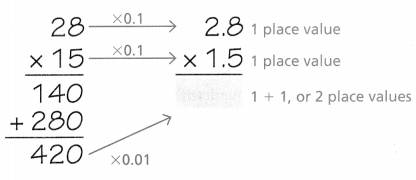So, the length of a male elephant seal is about ___4.20___ meters.
The length of a male elephant seal is about  4.20 meters,

Explanation:
A male leopard seal is measured and has a length of 2.8 meters,
A male elephant seal is about 1.5 times as long.
The length of the male elephant seal is
Step 1:
Multiplying as with whole numbers,
Step 2:
Placing the decimal point,
Think: Tenths are being multiplied by tenths. Using the pattern 0.1 × 0.1,
Placing the decimal point so the value of the decimal is 0.01,So, the length of a male elephant seal is about 4.20 meters.

What if you multiplied 2.8 by 1.74? What would be the place value of the product?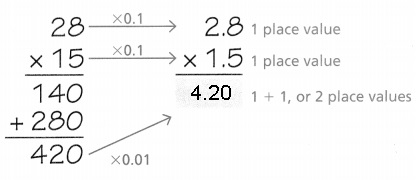The place value of the product is 0.01,

Explanation:
A male leopard seal is measured and has a length of 2.8 meters,
A male elephant seal is about 1.5 times as long.
The length of the male elephant seal is
Step 1:
Multiplying as with whole numbers,
Step 2:
Placing the decimal point,
Thinking tenths are being multiplied by tenths.
Using the pattern 0.1 × 0.1,
Placing the decimal point so the value of the decimal is 0.01.

Another Way Use estimation

You can use an estimate to place the decimal point in a product.

Multiply. 7.8 × 3.12
STEP 1:
Estimate by rounding each factor to the nearest whole number.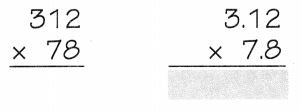STEP 2:
Multiplying as with whole numbers.

STEP 3:
Using the estimate to place the decimal point.
Think: The product should be close to your estimate.
7.8 × 3.12 = 24.336.
Upon multiplying 7.8 × 3.12 we get 24.336,Explanation:
Multiplying 7.8 × 3.12
STEP 1:
Estimate by rounding each factor to the nearest whole number,

STEP 2:
Multiplying as with whole numbers,

STEP 3:
Using the estimate to place the decimal point 7.8 × 3.12 = 24.336,
Thinking the product should be close to my estimate is 24.336 ≈ 24.

Share and Show

Place the decimal point in the product.

Question 1.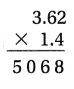Think: A hundredth is being multiplied by a tenth. Use the pattern 0.01 × 0.1.Multiplying 3.62 X 1.4 = 5.068,

Explanation:
Multiplying 3.62 × 1.4,
STEP 1:
Estimate by rounding each factor to the nearest whole number,
STEP 2:
Multiplying as with whole numbers,
362
X14
1448
3620
5068

STEP 3:
Using the estimate to place to the decimal point of 3.62 X 1.4 is 5.068
Thinking the product should be close to estimate is 4 X 1 ≈ 4.

Question 2.Estimate: 1 × 7 = ____7______Explanation:
Multiplying 6.8 × 1.2,
STEP 1:
Estimate by rounding each factor to the nearest whole number,
STEP 2:
Multiplying as with whole numbers,
68
X12
136
680
1
816

STEP 3:
Using the estimate to place to the decimal point as 6.8 X 1.2= 8.16,
Thinking that the product should be close to the estimate as 7 X 1 ≈ 7.

Find the product.

Question 3.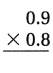Explanation:
Multiplying 0.9 × 0.8,
STEP 1:
Estimate by rounding each factor to the nearest whole number,
STEP 2:
Multiplying as with whole numbers,
09
X08
72

STEP 3:
Using the estimate to place to the decimal point as 0.9 X 0.8 = 0.72,
Thinking that the product should be close to the estimate as 1 X 1 ≈ 1.

Question 4.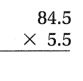Explanation:
Multiplying  84.5 × 5.5,
STEP 1:
Estimate by rounding each factor to the nearest whole number,
STEP 2:
Multiplying as with whole numbers,
845
X 55
04225
42250
46475

STEP 3:
Using the estimate to place to the decimal point as 84.5 X 5.5 =464.75
Thinking that the product should be close to the estimate as 85 X 5 ≈ 425.

Question 5.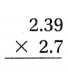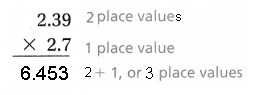Explanation:
Multiplying  2.39 × 2.7,
STEP 1:
Estimate by rounding each factor to the nearest whole number,
STEP 2:
Multiplying as with whole numbers,
239
X 27
1673
4780
6453

STEP 3:
Using the estimate to place to the decimal point as 2.39 X 2.7 = 6.453
Thinking that the product should be close to the estimate as 239 X 27 ≈ 6.453.

Math Talk
Mathematical Processes

Explain how you might know the place value of the product for Exercise 5 before you solve.
Place value of the product for Exercise 5 before solving is 3,

Explanation:
When multiplying  2.39 × 2.7,
we get place value from 2.39 as 2, because 39 is there after point(.) position,
therefore place value is 2 in 2.39,
now in 2.7, 7 is there after point(.) position, therefore place value is 1 in 2.7,
Totally there are 2 + 1 = 3 place values in 6.453 from 2.39 × 2.7.

Problem Solving

Practice: Copy and Solve Find the product.

Question 6.
3.4 × 5.2
3.4 X 5.2 = 17.68,
Place value of the product 3.4 X 5.2 is 2,

Explanation:
When multiplying  3.4 × 5.2,
we get place value from 3.4 as 1 because 4 is there after point(.) position,
therefore place value is 1 in 3.4,
now in 5.2 after point(.) position 2 is there, therefore place value is 1 in 5.2,
Totally there are 1 + 1 = 2 place values in 17.68 of 3.4 × 5.2.

Question 7.
0.9 × 2.46
0.9 X 2.46 = 2.214,
Place value of the product 0.9 X 2.46 is 2,

Explanation:
When multiplying  0.9 × 2.46,
we get place value from 0.9 as 1 because 9 is there after point(.) position,
therefore place value is 1 in 0.9,
now in 2.46 after point(.) position 2 is there, therefore place value is 2 in 2.46,
Totally there are 1 + 2 = 3 place values in 2.214.

Question 8.
9.1 × 5.7
9.1 X 5.7 = 51.87,
Place value of the product 9.1 X 5.7 is 2,

Explanation:
When multiplying  9.1 × 5.7,
we get place value from 9.1 as 1 because 9 is there after point(.) position,
therefore place value is 1 in 0.9,
now in 5.7 after point(.) position 7 is there, therefore place value is 1 in 5.7,
Totally there are 1 + 1 = 2 place values in 51.87.

Question 9.
4.8 × 6.01
4.8 X 6.01 = 51.870,
Place value of the product 4.8 X 6.01 is 3,

Explanation:
When multiplying  4.8 × 6.01,
we get place value from 4.8 as 1 because 8 is there after point(.) position,
therefore place value is 1 in 4.8,
now in 6.01 after point(.) position 01 is there, therefore place value is 2 in 6.01,
Totally there are 1 + 2 = 3 place values in 51.87.

Question 10.
7.6 × 18.7
7.6 X 18.7 = 51.87,
Place value of the product 7.6 X 18.7 is 2,

Explanation:
When multiplying  7.6 × 18.7,
we get place value from 7.6 as 1 because 6 is there after point(.) position,
therefore place value is 1 in 7.6,
now in 18.7 after point(.) position 01 is there, therefore place value is 1 in 18.7,
Totally there are 1 + 1 = 2 place values in 51.87.

Question 11.
1.5 × 9.34
1.5 X 9.34 = 14.010,
Place value of the product 1.5 X 9.34 is 3,

Explanation:
When multiplying 1.5 × 9.34,
we get place value from 1.5 as 1 because 5 is there after point(.) position,
therefore place value is 1 in 1.5,
now in 9.34 after point(.) position 34 is there, therefore place value is 2 in 9.34,
Totally there are 1 + 2 = 3 place values in 14.010.

Question 12.
0.77 × 14.9
1.5 X 9.34 = 14.010,
Place value of the product 1.5 X 9.34 is 3,

Explanation:
When multiplying 1.5 × 9.34,
we get place value from 1.5 as 1 because 5 is there after point(.) position,
therefore place value is 1 in 1.5,

now in 9.34 after point(.) position 34 is there, therefore place value is 2 in 9.34,
Totally there are 1 + 2 = 3 place values in 14.010.

Question 13.
3.3 × 58.14
3.3 X 58.14 = 191.862,
Place value of the product 3.3 X 58.14 is 3,

Explanation:
When multiplying 3.3 × 58.14,
we get place value from 3.3 as 1 because 3 is there after point(.) position,
therefore place value is 1 in 3.3,
now in 58.14 after point(.) position 2 is there, therefore place value is 2 in 58.14,
Totally there are 1 + 2 = 3 place values in 191.862.

Question 14.
H.O.T. Write Math In the multiplication problem 5.5 × 4.6, is the answer 25.3 or 25.30?
5.5 X 4.6 the answer is 25.3 and 25.30,

Explanation:
Given multiplication problem 5.5 X 4.6,
we get place value from 5.5 as 1 because 5 is there after point(.) position,
therefore place value is 1 in 5.5,

now in 4.6 after point(.) position 6 is there, therefore place value is 1 in 4.6,
Totally there are 1 + 1 = 2 place values in 5.5 X 4.6, therefore the answer is 25.30
and also 25.3 as zero has no value.

Problem Solving

Question 15.
Analyze Charlie has an adult Netherlands dwarf rabbit that weighs 1.2 kilograms.
Cliff’s adult Angora rabbit weighs 2.9 times as much as Charlie’s rabbit.
How much does Cliff’s rabbit weigh?
Cliff’s rabbit weigh 2.9 X 1.2 = 3.48 kilograms,

Explanation:
Given Charlie has an adult Netherlands dwarf rabbit that weighs 1.2 kilograms.
Cliff’s adult Angora rabbit weighs 2.9 times as much as Charlie’s rabbit so does
Cliff’s rabbit weigh 2.9 X 1.2 = 3.48 kilograms.

Question 16.
Multi-Step John has pet rabbits in an enclosure that has an area of 30.72 square feet.
The enclosure Taylor is planning to build for his rabbits will be 2.2 times as large as John’s.
How many more square feet will Taylor’s enclosure have than John’s enclosure?
67.584 square feet will Taylor’s enclose to have more than John’s enclosures,

Explanation:
Given John has pet rabbits in an enclosure that has an area of 30.72 square feet.
The enclosure Taylor is planning to build for his rabbits will be 2.2 times as large as John’s.
So more square feet will Taylor’s enclosure have than John’s enclosure is 30.72 X 2.2 = 67.584.

Question 17.
H.O.T. A  zoo is planning a new building for the penguin exhibit.
First, they made a model that was 1.3 meters tall. Then,
they made a more detailed model that was 1.5 times as tall as the first model.
The building will be 2.5 times as tall as the height of the detailed model.
What will be the height of the building?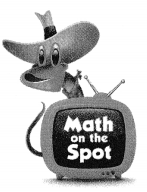The height of the new building was 0.78 meters,

Explanation:
Given a zoo is planning a new building for the penguin exhibit.
First, they made a model that was 1.3 meters tall. Then,
they made a more detailed model that was 1.5 times as tall as the first model.
So the detailed model is 1.3 x 1.5 = 1.95 meters tall,
The building will be 2.5 times as tall as the height of the detailed model.
So the height of the new building was 1.95 ÷ 2.5 = 0.78 meters.

Question 18.
Arnold is solving this multiplication problem: 8.12 × 4.7.
Which of these estimates can Arnold use to help him determine
where the decimal point belongs in the product?
(A) 80 × 40 = 3,200
(B) 8 × 5 = 40
(C) 800 × 4 = 3,200
(D) 800 × 5 = 4,000
(D) 800 × 5 = 4,000,

Explanation:
Arnold is solving this multiplication problem: 8.12 × 4.7.
So the estimates can Arnold use to help him determine
where the decimal point belongs in the product is as
8.12 has 2 decimal places value 12 so the nearest estimate of 812 is 800,
4.7 has 1 decimal place value 7 so the nearest estimate of 4.7 is 5,
therefore the total nearest estimate is 800 X 5 = 4,000 which matches with (D).

Question 19.
Which multiplication problem will not give the same product as 5.5 × 4.44?
(A) 5.5 × 4.44
(B) 0.55 × 44.4
(C) 55 × 0.444
(D) 0.55 × 0.444
(D) 0.55 × 0.444,

Explanation:
Multiplication problem will not give the same product as 5.5 × 4.44 is
5.5 has 1 decimal place value 5 and 4.44 has 2 decimal places value 44,
so the total decimal place values are 3,
Let’s check with (A) 5.5 X 4.44 which has total 3 place values,
Now with (B) 0.55 × 44.4 which has total 3 place values,
Now with (C) 55 × 0.444 which has total 3 place values,
Now with (D) 0.55 × 0.444 which has total 4 place values,
therefore, Answer is (D) will not give the same product as 5.5 × 4.44.

Question 20.
Multi-Step Gina bought 2.5 pounds of peaches that cost $1.38 per pound at the grocery store. Amy went to the local farmer’s market and purchased 3.5 pounds of peaches at$0.98 per pound.
Who spent more money, and how much more?
(A) Gina, $0.20 (B) Gina,$0.02
(C) Amy, $0.20 (D) Amy,$0.02
(B) Gina, $0.02, Explanation: Given Gina bought 2.5 pounds of peaches that cost$1.38 per pound at the grocery store,
So money spent is 2.5 X $1.38 =$3.45, Amy went to the local farmer’s market and
purchased 3.5 pounds of peaches at $0.98 per pound, So money spent is 3.5 X$0.98 = $3.43, On comparing Gina and Amy as$3.45 > $3.43 so (B) is true. Texas Test Prep Question 21. A vine in Mr. Jackson’s garden was 3.6 feet long. When it is measured again, it is 2.1 times as long. How long is the vine? (A) 7.5 feet (B) 6.6 feet (C) 5.7 feet (D) 7.56 feet Answer: (D) 7.56 feet, Explanation: Given a vine in Mr. Jackson’s garden was 3.6 feet long. When it is measured again, it is 2.1 times as long. So length of the vine is 3.6 X 2.1 = 7.56 feet, matches with 7.56. ### Texas Go Math Grade 5 Lesson 3.7 Homework and Practice Answer Key Find the product. Question 1. 0.6 × 0.8 Answer: 0.6 X 0.8 = 0.48, Explanation: When multiplying 0.6 × 0.8, we get place value from 0.6 as 1 because 6 is there after point(.) position, therefore place value is 1 in 0.6, now in 0.8 after point(.) position 8 is there, therefore place value is 1 in 0.8, Totally there are 1 + 1 = 2 place values in 0.48. Question 2. 18.2 × 2.2 Answer: 18.2 X 2.2 = 40.04, Explanation: When multiplying 18.2 × 2.2, we get place value from 18.2 as 1 because 2 is there after point(.) position, therefore place value is 1 in 18.2, now in 2.2 after point(.) position 2 is there, therefore place value is 1 in 2.2, Totally there are 1 + 1 = 2 place values in 40.48. Question 3. 3.84 × 4.5 Answer: 3.84 X 4.5 = 17.280, Explanation: When multiplying 3.84 × 4.5, we get place value from 3.84 as 2 because 84 is there after point(.) position, therefore place value is 2 in , now in 4.5 after point(.) position 5 is there, therefore place value is 1 in 4.5, Totally there are 2 + 1 = 3 place values in 17.280. Question 4. 1.9 × 4.3 Answer: 1.9 X 4.3 = 8.17, Explanation: When multiplying 1.9 × 4.3, we get place value from 1.9 as 1 because 9 is there after point(.) position, therefore place value is 1 in 1.9, now in 4.3 after point(.) position 3 is there, therefore place value is 1 in 4.3, Totally there are 1 + 1 = 2 place values in 8.17. Question 5. 0.7 × 2.51 Answer: 0.7 X 2.51 = 1.757, Explanation: When multiplying 0.7 × 2.51, we get place value from 0.7 as 1 because 7 is there after point(.) position, therefore place value is 1 in 0.7, now in 2.51 after point(.) position 51 is there, therefore place value is 1 in 2.51, Totally there are 1 + 2 = 3 place values in 1.757. Question 6. 6.2 × 3.2 Answer: 6.2 X 3.2 = 19.84, Explanation: When multiplying 6.2 × 3.2, we get place value from 6.2 as 1 because 2 is there after point(.) position, therefore place value is 1 in 6.2, now in 3.2 after point(.) position 2 is there, therefore place value is 1 in 3.2, Totally there are 1 + 1 = 2 place values in 19.84. Question 7. 6.6 × 4.01 Answer: 6.6 X 4.01 = 26.466, Explanation: When multiplying 6.6 X 4.01, we get place value from 6.6 as 1 because 6 is there after point(.) position, therefore place value is 1 in 6.6, now in 4.01 after point(.) position 01 is there, therefore place value is 2 in 4.01, Totally there are 1 + 2 = 3 place values in 26.466. Question 8. 5.7 × 13.4 Answer: 5.7 X 13.4 = 76.38, Explanation: When multiplying 5.7 × 13.4, we get place value from 5.7 as 1 because 7 is there after point(.) position, therefore place value is 1 in 5.7, now in 13.4 after point(.) position 4 is there, therefore place value is 1 in 13.4, Totally there are 1 + 1 = 2 place values in 76.38. Question 9. 1.3 × 5.54 Answer: 1.3 X 5.54 = 7.202, Explanation: When multiplying 1.3 X 5.54, we get place value from 1.3 as 1 because 3 is there after point(.) position, therefore place value is 1 in 1.3, now in 5.54 after point(.) position 54 is there, therefore place value is 2 in 5.54, Totally there are 1 + 2 = 3 place values in 7.202. Question 10. 2.4 × 8.35 Answer: 2.4 X 8.35 = 20.040, Explanation: When multiplying 2.4 X 8.35, we get place value from 2.4 as 1 because 4 is there after point(.) position, therefore place value is 1 in 2.4, now in 8.35 after point(.) position 35 is there, therefore place value is 2 in 8.35, Totally there are 1 + 2 = 3 place values in 20.040. Question 11. 0.55 × 16.3 Answer: 0.55 X 16.3 = 8.965, Explanation: When multiplying 0.55 X 16.3, we get place value from 0.55 as 2 because 55 is there after point(.) position, therefore place value is 2 in 0.55, now in 16.3 after point(.) position 3 is there, therefore place value is 1 in 16.3, Totally there are 2 + 1 = 3 place values in 8.965. Question 12. 4.7 × 38.12 Answer: 4.7 X 38.12 = 179.164, Explanation: When multiplying 4.7 X 38.12, we get place value from 4.7 as 1 because 7 is there after point(.) position, therefore place value is 1 in 4.7, now in 38.12 after point(.) position 12 is there, therefore place value is 2 in 38.12, Totally there are 1 + 2 = 3 place values in 179.164. Question 13. 8.9 × 7.6 Answer: 8.9 X 7.6 = 67.64, Explanation: When multiplying 8.9 × 7.6, we get place value from 8.9 as 1 because 9 is there after point(.) position, therefore place value is 1 in 8.9, now in 7.6 after point(.) position 6 is there, therefore place value is 1 in 7.6, Totally there are 1 + 1 = 2 place values in 67.64. Question 14. 24.3 × 0.8 Answer: 24.3 X 0.8 = 67.64, Explanation: When multiplying 24.3 × 0.8, we get place value from 24.3 as 1 because 9 is there after point(.) position, therefore place value is 1 in 8.9, now in 7.6 after point(.) position 6 is there, therefore place value is 1 in 7.6, Totally there are 1 + 1 = 2 place values in 67.64. Question 15. 7.07 × 12.3 Answer: 7.07 X 12.3 = 86.961, Explanation: When multiplying 7.07 X 12.3, we get place value from 7.07 as 2 because 07 is there after point(.) position, therefore place value is 2 in 7.07 , now in 12.3 after point(.) position 3 is there, therefore place value is 1 in 12.3, Totally there are 2 + 1 = 3 place values in 86.961. Question 16. 49.21 × 3.6 Answer: 49.21 X 3.6 = 177.156, Explanation: When multiplying 49.21 X 3.6, we get place value from 49.21as 2 because 21 is there after point(.) position, therefore place value is 2 in 49.21 , now in 3.3 after point(.) position 3 is there, therefore place value is 1 in 3.3, Totally there are 2 + 1 = 3 place values in 177.156. Problem Solving Question 17. The width of Mr. Simm’s driveway is 9.2 meters. The length is 3.4 times the width. What is the length of the driveway? Answer: 31.28 meters, Explanation: Given the width of Mr. Simm’s driveway is 9.2 meters. The length is 3.4 times the width, So the length of the driveway is 9.2 meters X 3.4 = 31.28 meters. Question 18. A female orangutan at the zoo weighs 79.3 pounds. A male orangutan weighs 2.3 times as much as a female orangutan. How much does the male orangutan weigh? Answer: The male orangutan weigh is 182.39 pounds, Explanation: A female orangutan at the zoo weighs 79.3 pounds, A male orangutan weighs 2.3 times as much as a female orangutan. The male orangutan weigh 79.3 X 2.3 = 182.39 pounds. Lesson Check Fill in the bubble completely to show your answer. Question 19. Javier uses estimation to check the product for 7.24 × 3.9. Which of the following is a reasonable estimate for the product? (A) 700 × 40 = 28,000 (B) 700 × 3 = 2,100 (C) 7 × 4 = 28 (D) 70 × 40 = 2,800 Answer: (C) 7 × 4 = 28, Explanation: Javier uses estimation to check the product for 7.24 × 3.9, The following is a reasonable estimate for the product as 7.24 is approximately equal to 7 and 3.9 is approximately equal to 4 so 7 X 4 =28. Question 20. Which multiplication problem will give the same product as 4.6 × 5.2? (A) 0.46 × 52 (B) 4.6 × 0.52 (C) 46 × 5.2 (D) 0.46 × 5.2 Answer: (A) 0.46 × 52, Explanation: Given multiplication problem as 4.6 X 5.2 we get 23.92, so checking with (A) 0.46 × 52 we get product as 23.92, with (B) 4.6 X 0.52 we get product as 2.392, with (C) 46 X 5.2 we get product as 239.2, with (D) 0.46 X 5.2 we get product as 2.392, therefore given problem matches with (A) 0.46 × 52. Question 21. Mrs. Jones plants two evergreen trees in her yard. The first tree is 6.25 feet tall. The second tree is 0.5 times as tall. How tall is the second tree? (A) 3.255 feet (B) 6.125 feet (C) 3.125 feet (D) 6.750 feet Answer: (C) 3.125 feet, Explanation: Given Mrs. Jones plants two evergreen trees in her yard. The first tree is 6.25 feet tall and the second tree is 0.5 times as tall as first tree, therefore the second evergreen tree is 3.125 feet tall matches with (C). Question 22. Mr. Endo buys 13.6 gallons of gasoline. He pays$3.95 per gallon.
How much does Mr. Endo spend on gasoline?
(A) $53.72 (B)$17.55
(C) $9.65 (D)$39.00
(A) $53.72, Explanation: Given Mr.Endo buys 13.6 gallons of gasoline, He pays$3.95 per gallon.
So does Mr. Endo spend on gasoline 13.6 X $3.95 =$53.72 matches with (C).

Question 23.
Multi-Step Maria makes a quilt with a width of 2.7 meters.
The length is 3.2 times the width. The area of a rectangle is the
length times the width of the rectangle. What is the area of Maria’s quilt?
(A) 8.64 square meters
(B) 23.328 square meters
(C) 5.90 square meters
(D) 27.648 square meters
(A) 8.64 square meters,

Explanation:
Given Maria makes a quilt with a width of 2.7 meters.
The length is 3.2 times the width. So length of the quilt is
2.7 meters X 3.2 = 8.64 meters, As the area of a rectangle is
the length times the width of the rectangle,
therefore the area of Maria’s quilt is (A) 8.64 square meters.

Question 24.
Multi-Step At the butcher shop, Jason bought 2.4 pounds of
ground beef for $5.20 per pound. He bought 3.2 pounds of ground turkey for$2.90 per pound. How much more did Jason spend on beef than on turkey?
(A) $3.20 (B)$2.30
(C) $21.76 (D)$9.58
(A) $3.20, Explanation: Given at the butcher shop, Jason bought 2.4 pounds of ground beef for$5.20 per pound.
Jason bought ground beef for 2.4 X $5.20 =$12.48 ,
he bought 3.2 pounds of ground turkey for $2.90 per pound, so ground turkey Jason bought for 3.2 X$2.90 = $9.28, therefore more did Jason spend on beef than on turkey is$12.48 – $9.28 =$3.2 matches with (A).